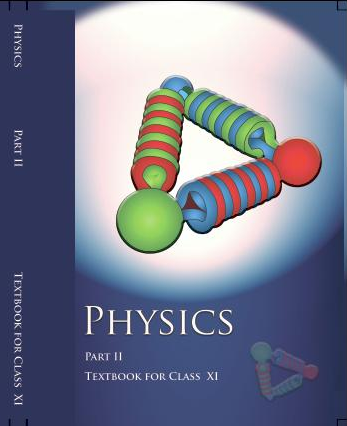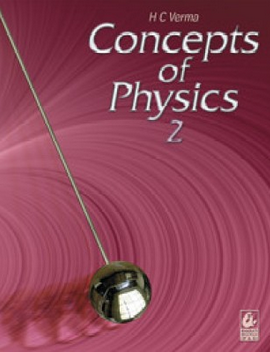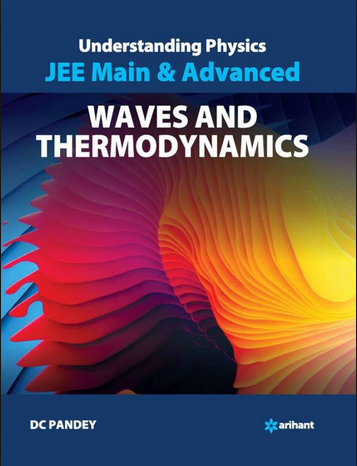Kinetic theory of Gases   Share

What is Kinetic theory of Gases

All of you must have seen hot air balloons in the sky or on television. The balloon rises because the air molecules get heated up and they started rising upwards and this heat (high temperature) increases the movement of molecules and the Kinetic energy of molecules increases hence resulting in a force which pushes the walls of the balloon in the upward direction. If you give keen attention to the above example you could see that the Temperature is related to Pressure. In fact, Temperature is directly proportional to Pressure and you can also see that balloon inflation also indicates the increase in Volume. In this chapter, we will see how these macroscopic properties i.e. Temperature, Pressure, and Volume, etc are related to each other.Kinetic Theory Of Gases- Topics

Equation of state for the perfect gas, work done while compressing a gas,Kinetic theory of gases (assumptions), concept of pressure, temperature and Kinetic energy , RMS speed of gas molecules; Degrees of freedom, applications to specific heat capacities of gases,Law of equipartition of energy,Avogadro's number,Mean free path.

Overview of Kinetic Theory of Gases

Before starting this chapter, you should know that it is comparatively easier topic than studying properties of solids or liquids because, in a gas, molecules are far from each other and their mutual interactions are negligible except when two molecules collide. Also, this chapter is going to be important because the next chapter you will be reading is Thermodynamics and many concepts of this chapter will be used there. This chapter mainly talks about the properties of ideal gases, and an ideal gas follows some set of assumption and also follows ideal gas equation which is given by, PV =nRT where P is the pressure in the container in which the gas is kept, V is the volume of the container, T is the temperature of gas, n is the number of moles of gas and R is a gas constant whose value is fixed.

The assumptions of the ideal gas are mentioned below for your better understanding of this chapter :

• The gas consists of a large number of molecules, which are in random motion and obey Newton's laws of motion;

• The volume of the molecules is negligibly small compared to the volume occupied by the gas

• No forces act on the molecules except during elastic collisions of negligible duration.

Prepare Online for JEE Main/NEET

Crack JEE 2021 with JEE/NEET Online Preparation Program

Although no real gas follows these assumptions hence they also don't follow the ideal gas equation. For real gases, some changes are made in the ideal gas equation. But for the intermediate class, the syllabus is limited to ideal gases only. You will also read about the Law of equipartition of energy which states that in equilibrium, the total energy is equally distributed in all possible energy modes, with each mode having average energy equal to  where  is Boltzmann’s constant. This law will also help you to understand the concept of degrees of freedom. You will get various questions in which you have to deal with Root mean square velocity, Average speed and Most probable speed of a molecule of gas. It also tells us that the temperature of an ideal monatomic gas is proportional to the average kinetic energy of its atoms.

Formulas for Kinetic Theory of Gases

• Ideal gas equation, PV =nRT

• Pressure of ideal gas,   where 'n' is number density of molecules, 'm' is mass of the molecule,  is mean squared speed of molecules.

• Root mean squared speed,

• Mean free path length,  ,here d is the diameter of molecule.

How to prepare the Kinetic Theory of Gases

It is an easy chapter in exam point of view hence if you have good command over calculation then you will be able to solve most of the questions very quickly but you shouldn’t take it lightly. Sometimes questions are asked which are hypothetical in nature and will require you to apply concepts hence read carefully don’t just mug up. Also, some questions will be very easy but tricky. For example, you may be given the value of , n, and Volume of the container and from there you will have to calculate the pressure of an ideal gas, so here you need to use two formulas, one will be  formula and 2nd will be the ideal gas equation. So keep in mind how can you convert one equation to get the required value of an unknown variable and that's why you will require a lot of practice for doing these types of conversions.

Kinetic Theory of Gases - Notes

• Give attention to the questions which were previously asked in the exam for which you are preparing, and look at the concept which was applied to solve them.

• Rather than just reading online or from books, make your own notes and revise them.

• Give a significant number of mock tests and make note of your progress.

• Make a list of questions which you were not able to solve in the first attempt or you took a long time solving them, after a week or two solve them again.

Books for Kinetics Theory of Gases

Recommendation for this chapter would be to first go through NCERT book and solve questions after that you should move on to NCERT Exemplar book for a good hold on this chapter. If you want to test yourself for competitive exams, then you should read Understanding Physics by D.C. Pandey or you could also read and practice questions from Concepts of Physics by H.C. Verma.

Physics Chapter wise Notes For Engineering and Medical Exams

 Chapters No. Chapters Name Chapter 1 Physics and Measurement Chapter 2 Kinematics Chapter 3 Laws of motion Chapter 4 Work Energy and Power Chapter 5 Rotational Motion Chapter 6 Gravitation Chapter 7 Properties of Solids and Liquids Chapter 9 Thermodynamics Chapter 10 Oscillations and Waves Chapter 11 Electrostatics Chapter 12 Current Electricity Chapter 13 Magnetic Effects of Current and Magnetism Chapter 14 Electromagnetic Induction and Alternating currents Chapter 15 Electromagnetic Waves Chapter 16 Optics Chapter 17 Dual Nature of Matter and Radiation Chapter  18 Atoms And Nuclei Chapter 19 Electronic devices Chapter 20 Communication Systems Chapter 21 Experimental skills

Topics from Kinetic theory of Gases

• Kinetic theory of gases- assumptions, concept of pressure ( JEE Main, GUJCET Pharmacy, COMEDK UGET ) (13 concepts)
• Equation of state of a perfect gas, work done on compressing a gas ( JEE Main, GUJCET Pharmacy, COMEDK UGET ) (2 concepts)
• Kinetic energy and temperature:rms speed of gas molecules: Degreesof freedom ( JEE Main, GUJCET Pharmacy, COMEDK UGET ) (9 concepts)
• Applications to specific heat capacities of gases: Mean free path, Avogadro'snumber ( JEE Main, GUJCET Pharmacy, COMEDK UGET ) (8 concepts)
• States of matter ( JEE Main, GUJCET Pharmacy, COMEDK UGET ) (1 concepts)
• Ideal gas, its equation and laws of ideal gas ( JEE Main, GUJCET Pharmacy, COMEDK UGET ) (5 concepts)
• Real gas ( JEE Main, GUJCET Pharmacy, COMEDK UGET ) (1 concepts)
• Pressure of an ideal gas ( JEE Main, GUJCET Pharmacy, COMEDK UGET ) (1 concepts)
• Maxwell's law ( JEE Main, GUJCET Pharmacy, COMEDK UGET ) (3 concepts)
• Law of equipartition of energy ( JEE Main, GUJCET Pharmacy, COMEDK UGET ) (4 concepts)

Important Books for Kinetic theory of Gases

•••Exams
Articles
Questions